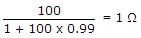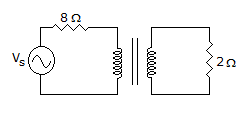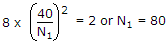# Electronics and Communication Engineering - Analog Electronics

41.

An amplifier has open loop gain of 100, input impedance 1 kΩ and output impedance 100 Ω. If negative feedback with β = 0.99 is used, the new input and output impedances are

 A. 10 Ω and 1 Ω B. 10 Ω and 10 kΩ C. 100 kΩ and 1 Ω D. 100 kΩ and 10 Ω

Explanation:

Input impedance with feedback = (1 kΩ) (1 + 100 + 0.99) = 100 kΩ. Output impedance with feedback =.

42.

In a BJT amplifier the power gain from base to collector is 4000. The power gain in dB is

 A. 3.6 B. 36 C. 7.2 D. 72

Explanation:

Gain in dB = 10 log (4000).

43.

In figure, secondary winding has 40 turns. For maximum power transformer to 2 ohm resistance the number of turns in primary isA. 20 B. 40 C. 80 D. 160

Explanation:.

44.

In a CE amplifier circuit the dc voltage between emitter and ground

 A. is always zero B. cannot be zero C. may or may not be zero D. is negative

Explanation:

Since dc is flowing through emitter resistance, dc voltage cannot be zero.

45.

A buffer amplifier should have

 A. high output impedance and high input impedance B. high output impedance and low input impedance C. low output impedance an low input impedance D. low output impedance and high input impedance